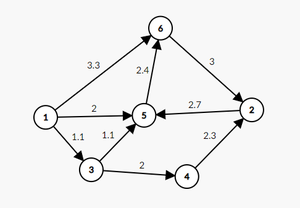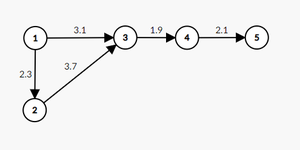GFG App
Open AppBrowser
Continue

# Monotonic shortest path from source to destination in Directed Weighted Graph

Given a weighted directed graph with N vertices and M edges, a source src and a destination target, the task is to find the shortest monotonic path (monotonically increasing or decreasing) from the source to the destination. Output -1 if no monotonic path is possible.

Note: All weights are non-negative

Examples:

Input: N = 6, M = 9, src = 1, target = 2
edges = {{1, 3, 1.1}, {1, 5, 2}, {1, 6, 3.3}, {2, 5, 2.7},
{3, 4, 2}, {3, 5, 1.1}, {4, 2, 2.3}, {5, 6, 2.4}, {6, 2, 3}}Graph for first example

Output: 5.4
Explanation: There are three monotonic paths in the graph
that originate from vertex 1, which are 1 – 6 – 2 because it is strictly increasing,
and 1 – 3 – 4 – 2, and 1 – 5 – 6 – 2 since both are strictly decreasing.
The shortest one of these paths is 1 – 3 – 4 – 2,
which has a sum of weights equal to 1.1 + 2 + 2.3 = 5.4,
So the output is 5.4.

Input: N = 5, M = 5, src = 1, target = 5
edges = {{1, 2, 2.3}, {1, 3, 3.1}, {2, 3, 3.7}, {3, 4, 1.9}, {4, 5, 2.1}}Graph for second example

Output: -1
Explanation: No monotonic path exists from vertex 1 to vertex 5.

Approach: To solve the problem follow the below idea:

Run Dijkstra’s algorithm twice: one for increasing shortest paths and another for decreasing shortest paths, and take the shorter path of the two results.

Follow the given steps to solve the problem:

• Run Dijkstra’s algorithm twice for both increasing and decreasing paths.
• While doing Dijkstra’s for decreasing shortest paths:
• Only update the shortest path to a vertex v from vertex u if the weight of the edge from u to v is less than the edge on the shortest path directed towards u
• Similarly for the increasing shortest paths:
• Only update the shortest path to a vertex v from u, if the edge from u to v is greater than the edge on the shortest path directed towards u.
• If the destination vertex has not yet been reached, then no valid shortest path exists.
• If both passes of Dijkstra’s on increasing and decreasing shortest paths result in no valid paths, then return -1.

Below is the implementation of the above approach.

## Java

 `import` `java.io.*;` `import` `java.util.*;`   `// Finds the monotonic shortest path` `// using Dijkstra's algorithm` `public` `class` `Main {` `    ``public` `static` `void` `main(String[] args)` `    ``{` `        ``int` `N = ``6``;` `        ``int` `M = ``9``;`   `        ``// Create an array of vertices` `        ``Vertex[] vertices = ``new` `Vertex[N + ``1``];`   `        ``// Create instances of each vertex from 1 to N` `        ``for` `(``int` `i = ``1``; i <= N; i++)` `            ``vertices[i] = ``new` `Vertex(i);`   `        ``vertices[``1``].adjList.add(``3``);` `        ``vertices[``1``].adjWeights.add(``1.1``);`   `        ``vertices[``1``].adjList.add(``5``);` `        ``vertices[``1``].adjWeights.add(``2.0``);`   `        ``vertices[``1``].adjList.add(``6``);` `        ``vertices[``1``].adjWeights.add(``3.3``);`   `        ``vertices[``2``].adjList.add(``5``);` `        ``vertices[``2``].adjWeights.add(``2.7``);`   `        ``vertices[``3``].adjList.add(``4``);` `        ``vertices[``3``].adjWeights.add(``2.0``);`   `        ``vertices[``3``].adjList.add(``5``);` `        ``vertices[``3``].adjWeights.add(``1.1``);`   `        ``vertices[``4``].adjList.add(``2``);` `        ``vertices[``4``].adjWeights.add(``2.3``);`   `        ``vertices[``5``].adjList.add(``6``);` `        ``vertices[``5``].adjWeights.add(``2.4``);`   `        ``vertices[``6``].adjList.add(``2``);` `        ``vertices[``6``].adjWeights.add(``3.0``);`   `        ``// Source and destination vertices` `        ``int` `src = ``1``;` `        ``int` `target = ``2``;` `        ``System.out.println(` `            ``shortestPath(vertices, N, src, target));` `    ``}`   `    ``public` `static` `double` `shortestPath(Vertex vertices[],` `                                      ``int` `N, ``int` `source,` `                                      ``int` `destination)` `    ``{` `        ``// Stores distance from source and edge` `        ``// on the shortest path from source` `        ``double``[] distTo = ``new` `double``[N + ``1``];` `        ``double``[] edgeTo = ``new` `double``[N + ``1``];`   `        ``// Set initial distance from source` `        ``// to the highest value` `        ``for` `(``int` `i = ``1``; i <= N; i++)` `            ``distTo[i] = Double.MAX_VALUE;`   `        ``// Monotonic decreasing pass of dijkstras` `        ``distTo = ``0.0``;` `        ``edgeTo = Double.MAX_VALUE;`   `        ``PriorityQueue pq` `            ``= ``new` `PriorityQueue(` `                ``new` `Comparator() {` `                    ``public` `int` `compare(Vertex a, Vertex b)` `                    ``{` `                        ``return` `Double.compare(distTo[a.id],` `                                              ``distTo[b.id]);` `                    ``}` `                ``});`   `        ``// Add the initial source vertex` `        ``// into the priority queue` `        ``pq.add(vertices);`   `        ``while` `(!pq.isEmpty()) {`   `            ``// Take the vertex with the closest` `            ``// current distance from source` `            ``Vertex closest = pq.remove();`   `            ``for` `(``int` `i = ``0``; i < closest.adjList.size();` `                 ``i++) {`   `                ``// Checks if the edges are decreasing and` `                ``// whether the current directed edge will` `                ``// create a shorter path` `                ``if` `(closest.adjWeights.get(i)` `                        ``< edgeTo[closest.id]` `                    ``&& distTo[closest.id]` `                               ``+ closest.adjWeights.get(i)` `                           ``< distTo[closest.adjList.get(` `                                 ``i)]) {` `                    ``edgeTo[closest.adjList.get(i)]` `                        ``= closest.adjWeights.get(i);` `                    ``distTo[closest.adjList.get(i)]` `                        ``= closest.adjWeights.get(i)` `                          ``+ distTo[closest.id];` `                    ``pq.add(` `                        ``vertices[closest.adjList.get(i)]);` `                ``}` `            ``}` `        ``}`   `        ``// Store the result of the first pass of dijkstras` `        ``double` `firstPass = distTo[destination];`   `        ``// Monotonic increasing pass of dijkstras` `        ``for` `(``int` `i = ``1``; i <= N; i++)` `            ``distTo[i] = Double.MAX_VALUE;` `        ``distTo = ``0.0``;` `        ``edgeTo = ``0.0``;`   `        ``// Add the initial source vertex` `        ``// into the priority queue` `        ``pq.add(vertices);`   `        ``while` `(!pq.isEmpty()) {`   `            ``// Take the vertex with the closest current` `            ``// distance from source` `            ``Vertex closest = pq.remove();`   `            ``for` `(``int` `i = ``0``; i < closest.adjList.size();` `                 ``i++) {`   `                ``// Checks if the edges are increasing and` `                ``// whether the current directed edge will` `                ``// create a shorter path` `                ``if` `(closest.adjWeights.get(i)` `                        ``> edgeTo[closest.id]` `                    ``&& distTo[closest.id]` `                               ``+ closest.adjWeights.get(i)` `                           ``< distTo[closest.adjList.get(` `                                 ``i)]) {` `                    ``edgeTo[closest.adjList.get(i)]` `                        ``= closest.adjWeights.get(i);` `                    ``distTo[closest.adjList.get(i)]` `                        ``= closest.adjWeights.get(i)` `                          ``+ distTo[closest.id];` `                    ``pq.add(` `                        ``vertices[closest.adjList.get(i)]);` `                ``}` `            ``}` `        ``}`   `        ``// Store the result of the second pass of Dijkstras` `        ``double` `secondPass = distTo[destination];`   `        ``if` `(firstPass == Double.MAX_VALUE` `            ``&& secondPass == Double.MAX_VALUE)` `            ``return` `-``1``;` `        ``return` `Math.min(firstPass, secondPass);` `    ``}` `}`   `// Represents a vertex in the graph` `// id stores the vertex number of the vertex instance` `// adjList stores the id's of adjacent vertices` `// adjWeights stores the weights of adjacent vertices with` `// the same indexing as adjList` `class` `Vertex {` `    ``int` `id;` `    ``ArrayList adjList;` `    ``ArrayList adjWeights;`   `    ``// A constructor which accepts` `    ``// the id of the vertex` `    ``public` `Vertex(``int` `num)` `    ``{` `        ``id = num;` `        ``adjList = ``new` `ArrayList();` `        ``adjWeights = ``new` `ArrayList();` `    ``}` `}`

## Python3

 `import` `heapq`   `class` `Vertex:` `    ``def` `__init__(``self``, ``id``):` `        ``self``.``id` `=` `id` `        ``self``.adjList ``=` `[]` `        ``self``.adjWeights ``=` `[]`   `def` `shortestPath(vertices, N, source, destination):` `    ``# Stores distance from source and edge` `    ``# on the shortest path from source` `    ``distTo ``=` `[``float``(``'inf'``) ``for` `_ ``in` `range``(N ``+` `1``)]` `    ``edgeTo ``=` `[``float``(``'inf'``) ``for` `_ ``in` `range``(N ``+` `1``)]`   `    ``# Set initial distance from source` `    ``# to the highest value` `    ``distTo ``=` `0.0` `    ``edgeTo ``=` `float``(``'inf'``)`   `    ``pq ``=` `[(``0``, source)]` `    ``heapq.heapify(pq)`   `    ``while` `pq:` `        ``_, closest ``=` `heapq.heappop(pq)`   `        ``for` `i ``in` `range``(``len``(vertices[closest].adjList)):`   `            ``# Checks if the edges are decreasing and` `            ``# whether the current directed edge will` `            ``# create a shorter path` `            ``if` `vertices[closest].adjWeights[i] < edgeTo[closest] ``and` `distTo[closest] ``+` `vertices[closest].adjWeights[i] < distTo[vertices[closest].adjList[i]]:` `                ``edgeTo[vertices[closest].adjList[i]] ``=` `vertices[closest].adjWeights[i]` `                ``distTo[vertices[closest].adjList[i]] ``=` `distTo[closest] ``+` `vertices[closest].adjWeights[i]` `                ``heapq.heappush(pq, (distTo[vertices[closest].adjList[i]], vertices[closest].adjList[i]))`   `    ``return` `distTo[destination]`   `N ``=` `6` `M ``=` `9`   `# Create an array of vertices` `vertices ``=` `[Vertex(i) ``for` `i ``in` `range``(``1``, N``+``1``)]`   `vertices[``0``].adjList.append(``2``)` `vertices[``0``].adjWeights.append(``1.1``)`   `vertices[``0``].adjList.append(``4``)` `vertices[``0``].adjWeights.append(``2.0``)`   `vertices[``0``].adjList.append(``5``)` `vertices[``0``].adjWeights.append(``3.3``)`   `vertices[``1``].adjList.append(``4``)` `vertices[``1``].adjWeights.append(``2.7``)`   `vertices[``2``].adjList.append(``3``)` `vertices[``2``].adjWeights.append(``2.0``)`   `vertices[``2``].adjList.append(``4``)` `vertices[``2``].adjWeights.append(``1.1``)`   `vertices[``3``].adjList.append(``1``)` `vertices[``3``].adjWeights.append(``2.3``)`   `vertices[``4``].adjList.append(``5``)` `vertices[``4``].adjWeights.append(``2.4``)`   `vertices[``5``].adjList.append(``1``)` `vertices[``5``].adjWeights.append(``3.0``)`   `# Source and destination vertices` `src ``=` `1` `target ``=` `2` `print``(shortestPath(vertices, N, src, target))`

## Javascript

 `class Vertex {` `  ``constructor(id) {` `    ``this``.id = id;` `    ``this``.adjList = [];` `    ``this``.adjWeights = [];` `  ``}` `}`   `function` `shortestPath(vertices, N, source, destination) {` `  ``// Stores distance from source and edge` `  ``// on the shortest path from source` `  ``let distTo = ``new` `Array(N + 1).fill(Infinity);` `  ``let edgeTo = ``new` `Array(N + 1).fill(Infinity);`   `  ``// Set initial distance from source` `  ``// to the highest value` `  ``distTo = 0.0;` `  ``edgeTo = Infinity;`   `  ``let pq = [[0, source]];` `  ``pq.sort((a, b) => a - b);`   `  ``while` `(pq.length > 0) {` `    ``let [_, closest] = pq.shift();`   `    ``for` `(let i = 0; i < vertices[closest].adjList.length; i++) {`   `      ``// Checks if the edges are decreasing and` `      ``// whether the current directed edge will` `      ``// create a shorter path` `      ``if` `(vertices[closest].adjWeights[i] < edgeTo[closest] && distTo[closest] + vertices[closest].adjWeights[i] < distTo[vertices[closest].adjList[i]]) {` `        ``edgeTo[vertices[closest].adjList[i]] = vertices[closest].adjWeights[i];` `        ``distTo[vertices[closest].adjList[i]] = distTo[closest] + vertices[closest].adjWeights[i];` `        ``pq.push([distTo[vertices[closest].adjList[i]], vertices[closest].adjList[i]]);` `        ``pq.sort((a, b) => a - b);` `      ``}` `    ``}` `  ``}`   `  ``return` `distTo[destination];` `}`   `let N = 6;` `let M = 9;`   `// Create an array of vertices` `let vertices = [];` `for` `(let i = 1; i <= N; i++) {` `  ``vertices.push(``new` `Vertex(i));` `}`   `vertices.adjList.push(2);` `vertices.adjWeights.push(1.1);`   `vertices.adjList.push(4);` `vertices.adjWeights.push(2.0);`   `vertices.adjList.push(5);` `vertices.adjWeights.push(3.3);`   `vertices.adjList.push(4);` `vertices.adjWeights.push(2.7);`   `vertices.adjList.push(3);` `vertices.adjWeights.push(2.0);`   `vertices.adjList.push(4);` `vertices.adjWeights.push(1.1);`   `vertices.adjList.push(1);` `vertices.adjWeights.push(2.3);`   `vertices.adjList.push(5);` `vertices.adjWeights.push(2.4);`   `vertices.adjList.push(1);` `vertices.adjWeights.push(3.0);`   `// Source and destination vertices` `let src = 1;` `let target = 2;` `console.log(shortestPath(vertices, N, src, target));`

## C++

 `#include ` `#include ` `#include ` `#include `   `using` `namespace` `std;`   `// Represents a vertex in the graph` `class` `Vertex {` `public``:` `    ``int` `id;` `    ``vector<``int``> adjList;` `    ``vector<``double``> adjWeights;`   `    ``// A constructor which accepts the id of the vertex` `    ``Vertex(``int` `num)` `        ``: id(num)` `    ``{` `    ``}` `};`   `// Finds the monotonic shortest path using Dijkstra's` `// algorithm` `double` `shortestPath(vector& vertices, ``int` `source,` `                    ``int` `destination)` `{` `    ``int` `N = vertices.size() - 1;`   `    ``// Stores distance from source and edge on the shortest` `    ``// path from source` `    ``vector<``double``> distTo(N + 1,` `                          ``numeric_limits<``double``>::max());` `    ``vector<``double``> edgeTo(N + 1,` `                          ``numeric_limits<``double``>::max());`   `    ``// Set initial distance from source to the highest value` `    ``for` `(``int` `i = 1; i <= N; i++) {` `        ``distTo[i] = numeric_limits<``double``>::max();` `    ``}`   `    ``// Monotonic decreasing pass of Dijkstra's` `    ``distTo = 0.0;` `    ``edgeTo = numeric_limits<``double``>::max();`   `    ``priority_queue,` `                   ``vector >,` `                   ``greater > >` `        ``pq;` `    ``pq.push(make_pair(0.0, source));`   `    ``while` `(!pq.empty()) {` `        ``// Take the vertex with the closest current distance` `        ``// from source` `        ``pair<``double``, ``int``> top = pq.top();` `        ``pq.pop();` `        ``int` `closest = top.second;`   `        ``for` `(``int` `i = 0;` `             ``i < vertices[closest].adjList.size(); i++) {` `            ``int` `neighbor = vertices[closest].adjList[i];` `            ``double` `weight = vertices[closest].adjWeights[i];`   `            ``// Checks if the edges are decreasing and` `            ``// whether the current directed edge will create` `            ``// a shorter path` `            ``if` `(weight < edgeTo[closest]` `                ``&& distTo[closest] + weight` `                       ``< distTo[neighbor]) {` `                ``edgeTo[neighbor] = weight;` `                ``distTo[neighbor] = distTo[closest] + weight;` `                ``pq.push(` `                    ``make_pair(distTo[neighbor], neighbor));` `            ``}` `        ``}` `    ``}`   `    ``// Store the result of the first pass of Dijkstra's` `    ``double` `firstPass = distTo[destination];`   `    ``// Monotonic increasing pass of Dijkstra's` `    ``for` `(``int` `i = 1; i <= N; i++) {` `        ``distTo[i] = numeric_limits<``double``>::max();` `    ``}`   `    ``distTo = 0.0;` `    ``edgeTo = 0.0;`   `    ``pq.push(make_pair(0.0, source));`   `    ``while` `(!pq.empty()) {` `        ``// Take the vertex with the closest current distance` `        ``// from source` `        ``pair<``double``, ``int``> top = pq.top();` `        ``pq.pop();` `        ``int` `closest = top.second;`   `        ``for` `(``int` `i = 0;` `             ``i < vertices[closest].adjList.size(); i++) {` `            ``int` `neighbor = vertices[closest].adjList[i];` `            ``double` `weight = vertices[closest].adjWeights[i];`   `            ``// Checks if the edges are increasing and` `            ``// whether the current directed edge will create` `            ``// a shorter path` `            ``if` `(weight > edgeTo[closest]` `                ``&& distTo[closest] + weight` `                       ``< distTo[neighbor]) {` `                ``edgeTo[neighbor] = weight;` `                ``distTo[neighbor] = distTo[closest] + weight;` `                ``pq.push(` `                    ``make_pair(distTo[neighbor], neighbor));` `            ``}` `        ``}` `    ``}`   `    ``// Store the result of the second pass of Dijkstras` `    ``double` `secondPass = distTo[destination];`   `    ``if` `(firstPass == DBL_MAX && secondPass == DBL_MAX)` `        ``return` `-1;`   `    ``return` `min(firstPass, secondPass);` `}`   `// Driver Code` `int` `main()` `{` `    ``int` `N = 6, M = 9, src, target;`   `    ``// Create N vertices, numbered 1 to N` `    ``vector vertices(N + 1, Vertex(0));`   `    ``for` `(``int` `i = 1; i <= N; i++) {` `        ``vertices[i] = Vertex(i);` `    ``}`   `    ``// Add M edges to the graph` `    ``vertices.adjList.push_back(3);` `    ``vertices.adjWeights.push_back(1.1);`   `    ``vertices.adjList.push_back(5);` `    ``vertices.adjWeights.push_back(2.0);`   `    ``vertices.adjList.push_back(6);` `    ``vertices.adjWeights.push_back(3.3);`   `    ``vertices.adjList.push_back(5);` `    ``vertices.adjWeights.push_back(2.7);`   `    ``vertices.adjList.push_back(4);` `    ``vertices.adjWeights.push_back(2.0);`   `    ``vertices.adjList.push_back(5);` `    ``vertices.adjWeights.push_back(1.1);`   `    ``vertices.adjList.push_back(2);` `    ``vertices.adjWeights.push_back(2.3);`   `    ``vertices.adjList.push_back(6);` `    ``vertices.adjWeights.push_back(2.4);`   `    ``vertices.adjList.push_back(2);` `    ``vertices.adjWeights.push_back(3.0);`   `    ``// Source and destination vertices` `    ``src = 1;` `    ``target = 2;`   `    ``double` `shortest = shortestPath(vertices, src, target);`   `    ``cout << shortest << endl;`   `    ``return` `0;` `}`

Output

`5.4`

Time Complexity: O(N log(N) + M)
Auxiliary Space: O(N)

My Personal Notes arrow_drop_up